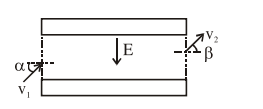# An electron with kinetic energyQuestion:

An electron with kinetic energy $K_{1}$ enters between parallel plates of a capacitor at an angle ' $\alpha$ ' with the plates. It leaves the plates at angle ' $\beta$ ' with kinetic energy $K_{2}$. Then the ratio of kinetic energies $K_{1}: K_{2}$ will be :

1. $\frac{\sin ^{2} \beta}{\cos ^{2} \alpha}$

2. $\frac{\cos ^{2} \beta}{\cos ^{2} \alpha}$

3. $\frac{\cos \beta}{\cos \alpha}$

4. $\frac{\cos \beta}{\sin \alpha}$

Correct Option: , 2

Solution:velocity along the plate will not change.

$\therefore v_{1} \cos \alpha=v_{2} \cos \beta$

$\frac{K_{1}}{K_{2}} \Rightarrow \frac{v_{1}^{2}}{v_{2}^{2}}=\frac{\cos ^{2} \beta}{\cos ^{2} \alpha}$